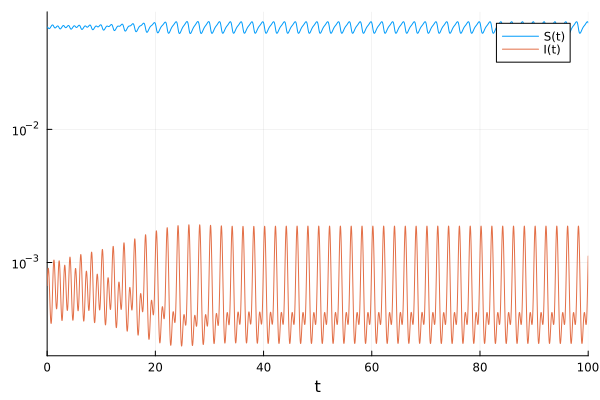# Initial conditions as functions of parameters in ModelingToolkit?

Hi everyone,

Is it possible to have initial conditions as functions of the parameters in ModelingToolkit?

IIRC, yes. That was discussed and added? It would be good to have it in an example or tutorial though.

Hmm, it appears to ‘just work’ now that I tried the obvious thing``````using ModelingToolkit
using OrdinaryDiffEq
using Plots

@parameters t R₀ γ μ a
@variables S(t) I(t) β(t)
D = Differential(t)

eqs = [D(S) ~ μ - β*S*I - μ*S,
D(I) ~ β*S*I - (γ+μ)*I,
β ~ R₀*(γ+μ)*(1+a*cos(2*π*t))]

@named sys = ODESystem(eqs)
simpsys = structural_simplify(sys)

S₀ = 1/R₀
I₀ = (μ/(μ+γ))*(1-S₀)
u₀ = [S => S₀, I => I₀]
p = [μ => 0.02, γ => 28.08, R₀ => 17.0, a => 0.08]
tspan = (0.0, 100.0)

prob = ODEProblem(simpsys, u₀, tspan, p)
sol = solve(prob, Rosenbrock23())

plot(sol, yscale=:log10)
``````1 Like